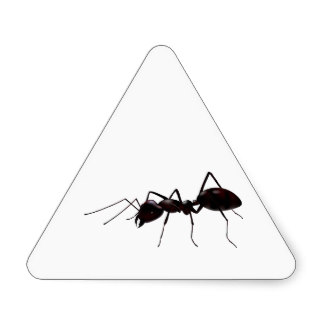# An ant on a triangle

Probability Level 4An ant is on the vertex of a triangle. It can move 'left' with probability of $\frac{1}{2}$ and 'right' with probability of $\frac{1}{2}$. Note that, the meaning of "moving left or right" is on the corresponding triangle's sides. The expected number of moves till it first reaches its starting point is ${\dfrac{a}{b}}$, where ${a}$ and ${b}$ are positive coprime integers. Calculate ${a+b}$.

×